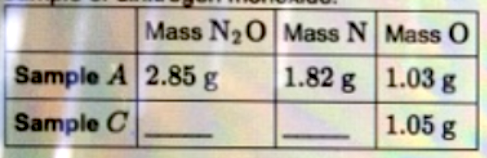# Problem: Use the law of constant composition to complete the following table summarizing the amounts of nitrogen and oxygen produced upon the sample of dinitrogen monoxide. Enter your answers numerically separated by a comma.

🤓 Based on our data, we think this question is relevant for Professor Scerri's class at UCLA.

###### FREE Expert Solution###### Problem Details

Use the law of constant composition to complete the following table summarizing the amounts of nitrogen and oxygen produced upon the sample of dinitrogen monoxide.What scientific concept do you need to know in order to solve this problem?

Our tutors have indicated that to solve this problem you will need to apply the Atomic Theory concept. You can view video lessons to learn Atomic Theory. Or if you need more Atomic Theory practice, you can also practice Atomic Theory practice problems.

What is the difficulty of this problem?

Our tutors rated the difficulty ofUse the law of constant composition to complete the followin...as high difficulty.

How long does this problem take to solve?

Our expert Chemistry tutor, Dasha took 4 minutes and 27 seconds to solve this problem. You can follow their steps in the video explanation above.

What professor is this problem relevant for?

Based on our data, we think this problem is relevant for Professor Scerri's class at UCLA.